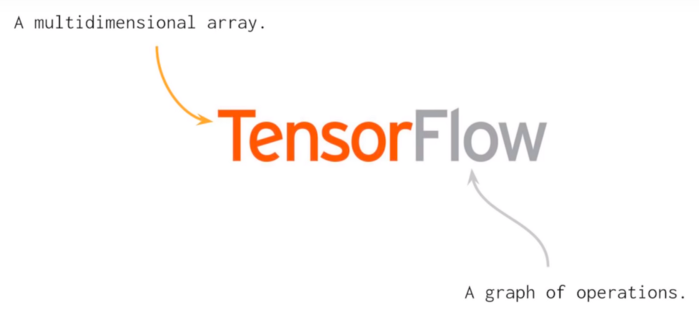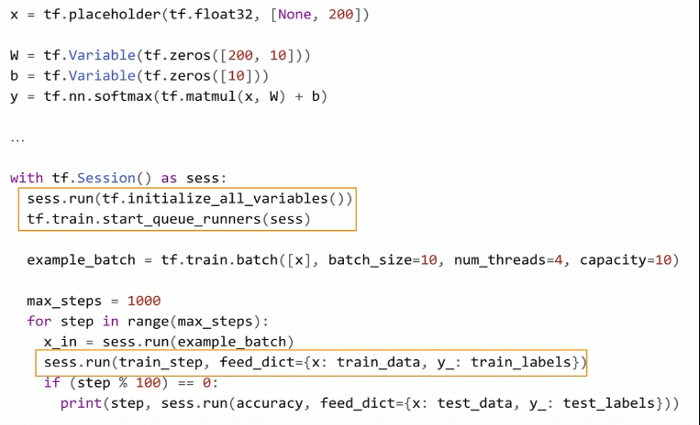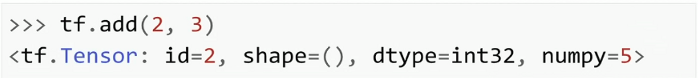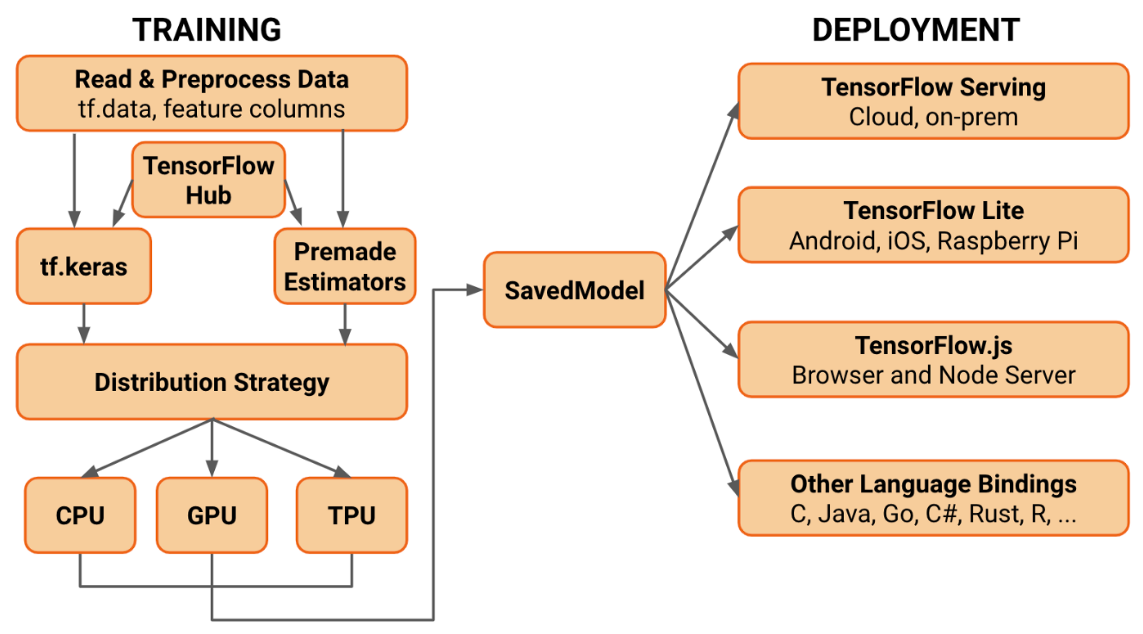# 关于 TensorFlow 2.0 你需要了解的一切

• 2019 年 7 月 15 日
• 本文字数：6741 字

阅读完需：约 22 分钟2019 年 6 月 26 日，在 Sao Paulo 举行的PAPIs.io LATAM会议上，作为Daitan的代表，本文作者Thalles Silva举办了一个关于 TensorFlow（TF）2.0 的研讨会，并在会上探讨了一些关于 TF 2.0 的话题。研讨会的初衷是重点展示 2.0 版本同以往 1.x 版本的不同。本文回顾了会上讨论的主要内容：Keras-APIs、SavedModels、TensorBoard、Keras-Tuner 等。同时，你也可以通过 Colab notebook 来查看练习代码。

# TensorFlow 2.0 简介

TensorFlow 是谷歌在 2015 年开源的一个通用高性能计算库。从一开始，TensorFlow 的主要目的就是为构建神经网络（NN）提供高性能 API。然而，借助于机器学习（ML）社区对它的兴趣以及时间上的优势，这个类库演变成了一个完整的 ML 生态系统。# 即时执行（Eager Execution）变为默认模式TensorFlow 1.x 代码示例TensorFlow 2.0 代码示例

TensorFlow 2.0 的代码看起来非常像 NumPy 代码。实际上，TensorFlow 和 NumPy 的对象也可以很容易地相互置换。因此，你也不用为 placeholders、Sessions 以及 feed_dictionaties 等伤脑筋了。

# API 清理

TensorFlow 2.0 中，tf.keras 是推荐使用的高级 API

# 初级 API

model = tf.keras.models.Sequential()model.add(Flatten(input_shape=(IMAGE_HEIGHT,IMAGE_WIDTH)))model.add(Dense(units=32, activation='relu'))model.add(Dropout(0.3))model.add(Dense(units=32, activation='relu'))model.add(Dense(units=10, activation='softmax'))# Configures the model for training.# Define the model optimizer, the loss function and the accuracy metricsmodel.compile(optimizer='adam',              loss='sparse_categorical_crossentropy',              metrics=['accuracy'])model.summary()

# Keras-Tuner

Keras-Tuner是一个专门为 Keras 模型优化超参数的独立库。在本文写作的时候，该类库仍处于 pre-alpha 阶段，即便如此，它已经可以在 Colab 上很好地同 tf.keras 以及 TensorFlow2.0 beta 一起工作。

def build_model(hp):    # define the hyper parameter ranges for the learning rate, dropout and hidden unit    hp_units = hp.Range('units', min_value=32, max_value=128, step=32)    hp_lr = hp.Choice('learning_rate', values=[1e-2, 1e-3, 1e-4])    hp_dropout = hp.Choice('dropout', values=[0.1,0.2,0.3])      # build a Sequential model    model = keras.Sequential()    model.add(Flatten(input_shape=(IMAGE_HEIGHT,IMAGE_WIDTH)))    model.add(Dense(units=hp_units, activation='relu'))    model.add(Dropout(hp_dropout))    model.add(Dense(units=32, activation='relu'))    model.add(layers.Dense(10, activation='softmax'))      # compile and return the model    model.compile(optimizer=keras.optimizers.Adam(hp_lr),        loss='sparse_categorical_crossentropy',        metrics=['accuracy'])    return model      # create a Random Search tuner  tuner = RandomSearch(      build_model,      objective='val_accuracy', # define the metric to be optimized over      max_trials=3,      executions_per_trial=1,      directory='my_logs') # define the output log/checkpoints folder      # start hyper-parameter optmization search  tuner.search(x_train, y_train,               epochs=2,               validation_data=(x_test, y_test))

# 高级 API

class Model(tf.keras.Model):  def __init__(self):    # Define the layers here    super(Model, self).__init__()    self.conv1 = Conv2D(filters=8, kernel_size=4, padding="same", strides=1, input_shape=(IMAGE_HEIGHT,IMAGE_WIDTH,IMAGE_DEPTH))    self.conv2 = Conv2D(filters=16, kernel_size=4, padding="same", strides=1)    self.pool = MaxPool2D(pool_size=2, strides=2, padding="same")    self.flat = Flatten()    self.probs = Dense(units=N_CLASSES, activation='softmax', name="output")    def call(self, x):    # Define the forward pass    net = self.conv1(x)    net = self.pool(net)    net = self.conv2(net)    net = self.pool(net)    net = self.flat(net)    net = self.probs(net)    return net    def compute_output_shape(self, input_shape):    # You need to override this function if you want to use the subclassed model    # as part of a functional-style model.    # Otherwise, this method is optional.    shape = tf.TensorShape(input_shape).as_list()    shape[-1] = self.num_classes    return tf.TensorShape(shape)

• 正向传播

• 损失函数评估

• 反向传播

• 梯度下降

# 配置 TensorBoard

 Load the TensorBoard notebook extension  %load_ext tensorboard    # create the tensorboard callback  tensorboard = TensorBoard(log_dir='logs/{}'.format(time.time()), histogram_freq=1)    # train the model  model.fit(x=x_train,             y=y_train,             epochs=2,             validation_data=(x_test, y_test),             callbacks=[tensorboard])    # launch TensorBoard  %tensorboard --logdir logs

• EarlyStopping：如同字面含义，它设置了一条规则，当被检测的量化值停止改善时就结束训练。

• ReduceLROnPlateau：当评估值停止改善的时候就降低学习率。

• TerminateOnNaN：当遇到一个 NaN 损失的时候就回调结束训练。

• LambdaCallback：一个创建样本的回调，自定义回调正在开发过程中。

# 提升 EagerCode 的性能

@tf.function  def train_step(images, labels):      with tf.GradientTape() as tape:      # forward pass      predictions = model(images)            # compute the loss      loss = cross_entropy(tf.one_hot(labels, N_CLASSES), predictions)        # get the gradients w.r.t the model's weights    gradients = tape.gradient(loss, model.trainable_variables)        # perform a gradient descent step    optimizer.apply_gradients(zip(gradients, model.trainable_variables))        # accumulates the training loss and accuracy    train_loss(loss)    train_accuracy(labels, predictions)

# 模型保存和恢复

TensorFlow 1.x 中缺少的另外一项标准就是我们如何为产品保存和装载模型。TensorFlow 2.0 试图通过定义一个单一 API 来解决这个问题。

TF 2.0 并没有采用保存模型的很多方法，它反而标准化了一个叫做 SavedModel 的抽象。

# serialize your model to a SavedModel object  # It includes the entire graph, all variables and weights  model.save('/tmp/model', save_format='tf')    # load your saved model  model = tf.keras.models.load_model('/tmp/model')

TensorFlow 生态系统整合了 SavedModels，也就是说，你可以将它部署到很多设备中，诸如手机、边缘设备以及服务器等。# TF-Lite 转化

# create a TensorFlow Lite converter   converter = tf.lite.TensorFlowLiteConverter.from_keras_model(model)    # performs model quantization to reduce the size of the model and improve latency  converter.optimizations = [tf.lite.Optimize.OPTIMIZE_FOR_SIZE]    tflite_model = converter.convert()

# TensorFLow.js 转化

!tensorflowjs_converter \      --input_format=tf_saved_model \      --saved_model_tags=serve \      --output_format=tfjs_graph_model \      /tmp/model \      /tmp/web_modelview raw

# 结语

2019 年 7 月 15 日 13:549326## 评论 1 条评论2019 年 07 月 17 日 09:13

• ###### 43 丨深度学习（下）：如何用 Keras 搭建深度学习网络做手写数字识别？

我们之前用KNN算过手写数字识别这个项目，这节课我们用Keras这个深度学习框架做一下，两者之间到底有什么区别呢？

2019 年 3 月 22 日

• ###### 都在关心 TensorFlow 2.0，那我手里基于 1.x 构建的程序怎么办？

本文将跟大家分享作者在处理TensorFlow适配和版本选择问题方面的经验，希望对你有所帮助。

• ###### 11｜深度学习（上）：用 CNN 带你认识深度学习

要构建出能够实现图像分类的CNN，我们通常需要把“卷积+池化”的组合重复搭建几次，形成深度卷积网络。

2021 年 9 月 22 日

• ###### Google 开源新 TensorFlow 运行时 TFRT，将取代现有运行时

最终，TFRT 将成为 TensorFlow 的默认运行时。

• ###### 25 丨 KNN（下）：如何对手写数字进行识别？

今天我用KNN、SVM、朴素贝叶斯和决策树做分类器，分别做一下手写数字分类识别的实战。

2019 年 2 月 8 日

• ###### 21 丨朴素贝叶斯分类（下）：如何对文档进行分类？

我们上一节讲了朴素贝叶斯的工作原理，今天我们来讲下这些原理是如何指导实际业务的。

2019 年 1 月 30 日

• ###### Introduction to the Keras Tuner

The Keras Tuner is a library that helps you pick the optimal set of hyperparameters for your TensorFlow program. The process of selecting the right set of hyperparameters for your machine learning (ML) application is called hyperparameter

2021 年 7 月 31 日

本文为下篇。

• ###### 技术解读：英特尔 x86 平台上，AI 能力是如何进行演进的？

AI 计算力的指数增长意味着，为了解决越来越复杂的用例，即使是 1000 倍的计算性能增长也很容易被消耗。因此，需要通过软件生态系统的助力，才能达到更好的性能。我们相信，构建 AI 软件生态系统，是将人工智能和数据科学项目推向生产的关键。

• ###### 分布式 tensorflow 源码解读 2：MonitoredTrainingSession

MonitoredTrainingSession是tensorflow管理分布式训练中一个使用很广泛的API

• ###### 如何信任你的深度学习代码？

深度学习是一门很难评估代码正确性的学科。

• ###### Inside Tensorflow 之：tf.keras part1

其实在Layer中也可以实现losses和metrics的追踪功能。

• ###### 机器学习排序算法：单点法排序学习

单点法排序学习是一类最简单也最实用的机器学习排序算法，在工业界得到广泛应用且效果显著。

2017 年 11 月 20 日

• ###### 编写复杂 TensorFlow 表达式的好工具：TF-Coder

TF-coder 是一款程序合成工具，可以帮助你编写 TensorFlow 代码。

• ###### 谷歌官方解读：TensorFlow 2.0 哪些新特性值得关注？

2018 年 11 月，TensorFlow 在庆祝 3 岁生日时回顾了其多年来增加的功能。 对于即将发布的 TensorFlow 2.0，TensorFlow 团队感到非常兴奋。相比于旧版本，这个被团队视为另一重要里程碑的版本又新增了哪些功能呢？

• ###### 18｜Wide&Deep：怎样让你的模型既有想象力又有记忆力？

Wide&Deep模型在业界有着巨大的影响力，只要掌握了它，我们就能抓住深度推荐模型这几年发展的一个主要方向。

2020 年 11 月 20 日

Java

hashmap

#### 互联网中的缓存